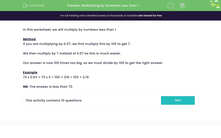# Multiply by Numbers Less Than 1

In this worksheet, students will multiply by numbers less than 1.Key stage:  KS 3

Curriculum topic:   Number

Curriculum subtopic:   Use Four Operations for All Numbers

Difficulty level:#### Worksheet Overview

In this activity, we will multiply by numbers less than 1.

Method

The easiest way to multiply using decimals is to count how many decimal places you have in your calculation and make sure that you have the same number in your answer.

For example, if you wanted to multiply 27 x 0.07 we have two decimal places - we've underlined them so you can see them easily!

Having counted them, we remove the decimal point and simply multiply the digits together: 27 x 7 = 189

Finally, we put the decimal point back in, making sure we still have two decimal places: 1.89

Pretty simple, right?

Here's one more example for you to look at:

42.65 x 0.006

First, count how many decimal places we have in 42.63 x 0.006    - we've underlined them again so you can spot them easily! There are five decimal places.

Remove the decimal point and do this calculation: 4263 x 6 = 25578

Finally, add the decimal point back in, making sure we have five decimal places: 0.25578

Let's have a go at some questions now

### What is EdPlace?

We're your National Curriculum aligned online education content provider helping each child succeed in English, maths and science from year 1 to GCSE. With an EdPlace account you’ll be able to track and measure progress, helping each child achieve their best. We build confidence and attainment by personalising each child’s learning at a level that suits them.

Get started